# loglog

Log-log scale plot

•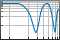## Syntax

``loglog(X,Y)``
``loglog(X,Y,LineSpec)``
``loglog(X1,Y1,...,Xn,Yn)``
``loglog(X1,Y1,LineSpec1,...,Xn,Yn,LineSpecn)``
``loglog(Y)``
``loglog(Y,LineSpec)``
``loglog(tbl,xvar,yvar)``
``loglog(tbl,yvar)``
``loglog(ax,___)``
``loglog(___,Name,Value)``
``p = loglog(___)``

## Description

### Vector and Matrix Data

example

````loglog(X,Y)` plots x- and y-coordinates using a base-10 logarithmic scale on the x-axis and the y-axis. To plot a set of coordinates connected by line segments, specify `X` and `Y` as vectors of the same length.To plot multiple sets of coordinates on the same set of axes, specify at least one of `X` or `Y` as a matrix. ```

example

````loglog(X,Y,LineSpec)` creates the plot using the specified line style, marker, and color.```

example

````loglog(X1,Y1,...,Xn,Yn)` plots multiple pairs of x- and y-coordinates on the same set of axes. Use this syntax as an alternative to specifying coordinates as matrices.```

example

````loglog(X1,Y1,LineSpec1,...,Xn,Yn,LineSpecn)` assigns specific line styles, markers, and colors to each x-y pair. You can specify `LineSpec` for some x-y pairs and omit it for others. For example, `loglog(X1,Y1,'o',X2,Y2)` specifies markers for the first x-y pair but not for the second pair.```

example

````loglog(Y)` plots `Y` against an implicit set of x-coordinates. If `Y` is a vector, the x-coordinates range from 1 to `length(Y)`.If `Y` is a matrix, the plot contains one line for each column in `Y`. The x-coordinates range from 1 to the number of rows in `Y`. If `Y` contains complex numbers, `loglog` plots the imaginary part of `Y` versus the real part of `Y`. However, if you specify both `X` and `Y`, MATLAB® ignores the imaginary part.```
````loglog(Y,LineSpec)` plots `Y` using implicit x-coordinates, and specifies the line style, marker, and color.```

### Table Data

example

````loglog(tbl,xvar,yvar)` plots the variables `xvar` and `yvar` from the table `tbl`. To plot one data set, specify one variable for `xvar` and one variable for `yvar`. To plot multiple data sets, specify multiple variables for `xvar`, `yvar`, or both. If both arguments specify multiple variables, they must specify the same number of variables. (since R2022a)```
````loglog(tbl,yvar)` plots the specified variable from the table against the row indices of the table. Timetables are not supported for this syntax. (since R2022a)```

example

````loglog(ax,___)` displays the plot in the target axes. Specify the axes as the first argument in any of the previous syntaxes.```

example

````loglog(___,Name,Value)` specifies `Line` properties using one or more name-value arguments. The properties apply to all the plotted lines. Specify the name-value arguments after all the arguments in any of the previous syntaxes. For a list of properties, see Line Properties.```

example

````p = loglog(___)` returns a `Line` object or an array of `Line` objects. Use `p` to modify properties of the plot after creating it. For a list of properties, see Line Properties.```

## Examples

collapse all

Define `x` as a vector of 50 logarithmically spaced numbers on the interval [${10}^{-1},{10}^{2}$]. Define `y` as ${2}^{\mathit{x}}$. Then plot `x` and `y`, and call the `grid` function to show the grid lines.

```x = logspace(-1,2); y = 2.^x; loglog(x,y) grid on```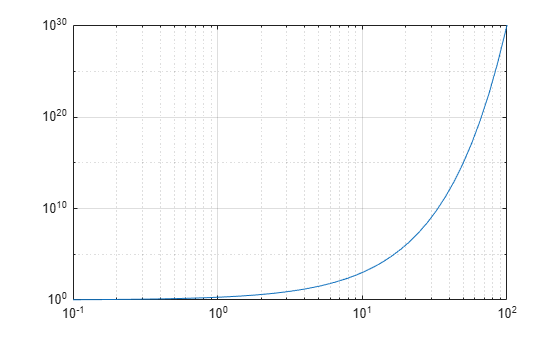Create a vector of x-coordinates and two vectors of y-coordinates. Plot two lines by passing comma-separated x-y pairs to `loglog`.

```x = logspace(-1,2); y1 = 10.^x; y2 = 1./10.^x; loglog(x,y1,x,y2) grid on```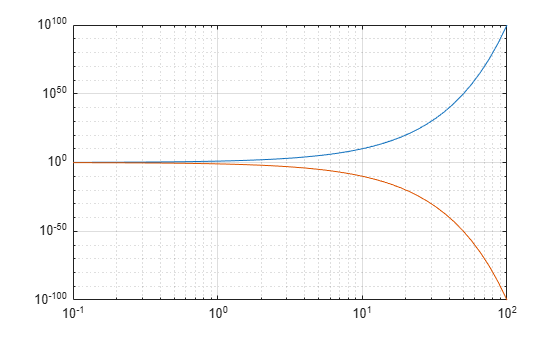Alternatively, you can create the same plot with one x-y pair by specifying y as a matrix: `loglog(x,[y1;y2])`.

Create a set of x- and y-coordinates and display them in a log-log plot.

```x = logspace(-1,2,10000); y = 5 + 3*sin(x); loglog(x,y)```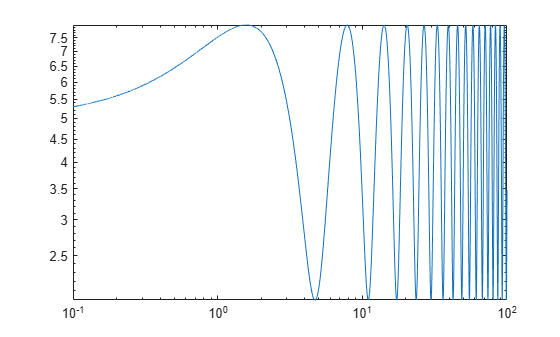Call the `yticks` function to position the y-axis tick values at whole number increments along the y-axis. Then create x- and y-axis labels by calling the `xlabel` and `ylabel` functions.

```yticks([3 4 5 6 7]) xlabel('x') ylabel('5 + 3 sin(x)')```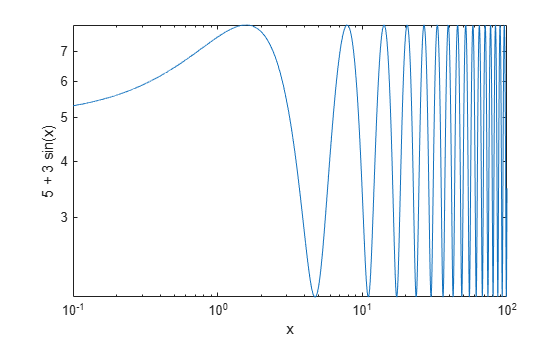Create a set of x- and y-coordinates and display them in a log-log plot. Specify the line style as `'s'` to display square markers without connecting lines. Specify the marker fill color as the RGB triplet `[0 0.447 0.741]`, which corresponds to a dark shade of blue.

```x = logspace(-1,2,20); y = 10.^x; loglog(x,y,'s','MarkerFaceColor',[0 0.447 0.741]) grid on```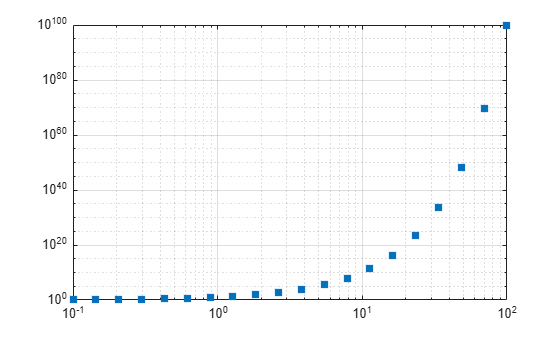Create two sets of x- and y-coordinates and display them in a log-log plot. Display a legend in the upper left corner of the plot by calling the `legend` function and specifying the location as `'northwest'`.

```x = logspace(-1,2,10000); y1 = 5 + 3*sin(x/4); y2 = 5 - 3*sin(x/4); loglog(x,y1,x,y2,'--') legend('Signal 1','Signal 2','Location','northwest')```When you specify only one coordinate vector, `loglog` plots those coordinates against the values `1:length(y)`. For example, define `y` as a vector of 6 values between `0.001` and `100`. Create a log-log plot of y.

```y = [0.001 0.01 0.1 1 10 100]; loglog(y) grid on```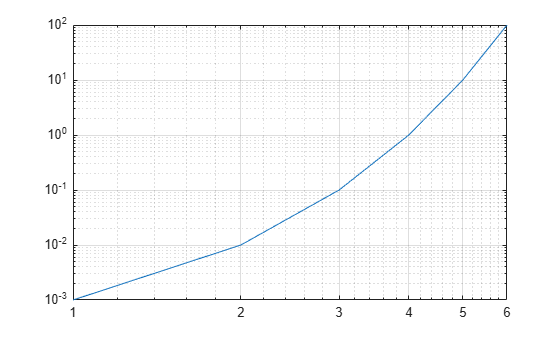If you specify `y` as a matrix, the columns of y are plotted against the values `1:size(y,1)`. For example, define `y` as a 5-by-3 matrix and pass it to the `loglog` function. The resulting plot contains 3 lines, each of which has x-coordinates that range from `1` to `5`.

```y = [0.0010 0.0100 0.1000 0.0100 0.1000 1.0000 0.1000 1.0000 10.0000 1.0000 10.5000 100.0000 10.0000 100.0000 1000.0000]; loglog(y) grid on```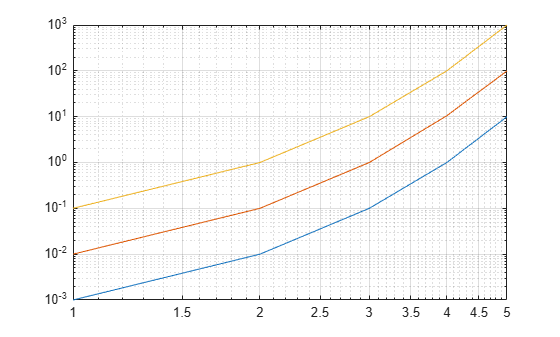Since R2022a

A convenient way to plot data from a table is to pass the table to the `loglog` function and specify the variables to plot.

Create a table containing two variables. Then display the first three rows of the table.

```Input = logspace(-1,2)'; Output = 10.^Input; tbl = table(Input,Output); head(tbl,3)```
``` Input Output _______ ______ 0.1 1.2589 0.11514 1.3036 0.13257 1.357 ```

Plot the `Input` variable on the x-axis and the `Output` variable on the y-axis. Return the `Line` object as `p`, and turn the axes grid on. Notice that the axis labels match the variable names.

```p = loglog(tbl,"Input","Output"); grid on```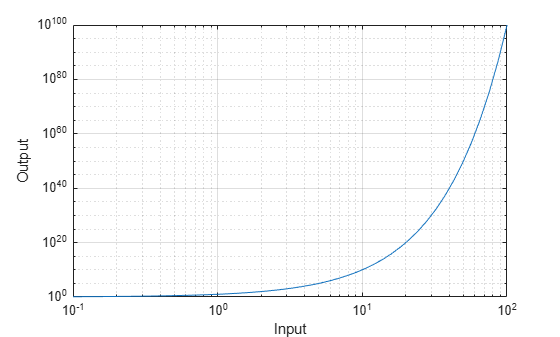To modify aspects of the line, set the `LineStyle`, `Color`, and `Marker` properties on the `Line` object. For example, change the line to a red dotted line with point markers.

```p.LineStyle = ":"; p.Color = "red"; p.Marker = ".";```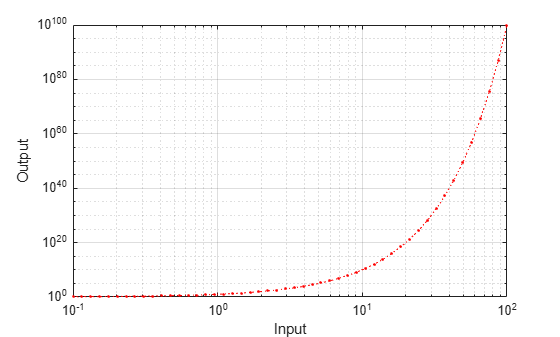Since R2022a

Create a table containing three variables. Then display the first three rows in the table.

```Input = logspace(-1,2)'; Output1 = 10.^Input; Output2 = 1./10.^Input; tbl = table(Input,Output1,Output2); head(tbl,3)```
``` Input Output1 Output2 _______ _______ _______ 0.1 1.2589 0.79433 0.11514 1.3036 0.76711 0.13257 1.357 0.73693 ```

Plot the `Input` variable on the x-axis and the `Output1` and `Output2` variables on the y-axis. Add a legend. Notice that the legend labels match the variable names.

```loglog(tbl,"Input",["Output1" "Output2"]) grid on legend```Create a tiled chart layout in the `'flow'` tile arrangement, so that the axes fill the available space in the layout. Next, call the `nexttile` function to create an axes object and return it as `ax1`. Then display a log-log plot by passing `ax1` to the `loglog` function.

```tiledlayout('flow') ax1 = nexttile; x = logspace(-1,2); y1 = 10.^x; loglog(ax1,x,y1)```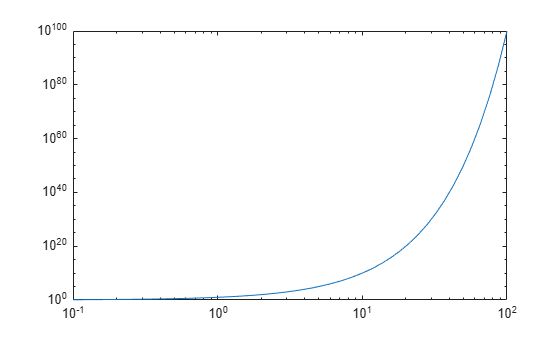Repeat the process to create a second axes object and a second log-log plot.

```ax2 = nexttile; y2 = 1./10.^x; loglog(ax2,x,y2)```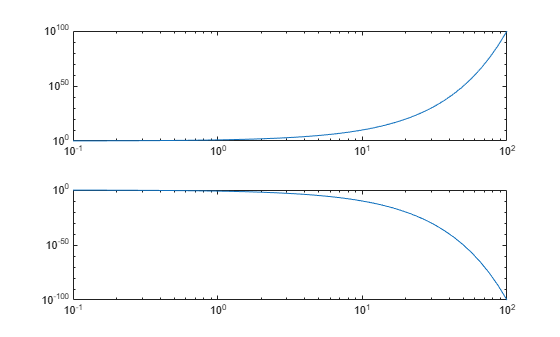Create a log-log plot containing two lines, and return the line objects in the variable `lg`.

```x = logspace(-1,2); y1 = 10.^x; y2 = 1./10.^x; lg = loglog(x,y1,x,y2);```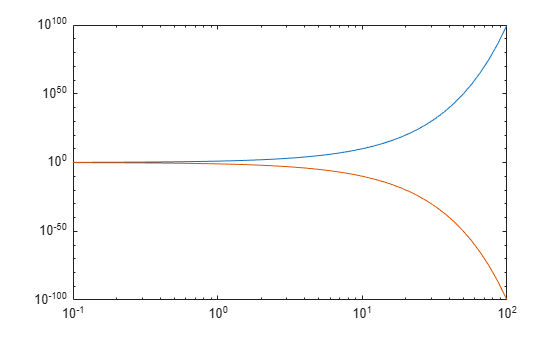Change the width of the first line to `2`, and change the color of the second line to purple.

```lg(1).LineWidth = 2; lg(2).Color = [0.4 0 1];```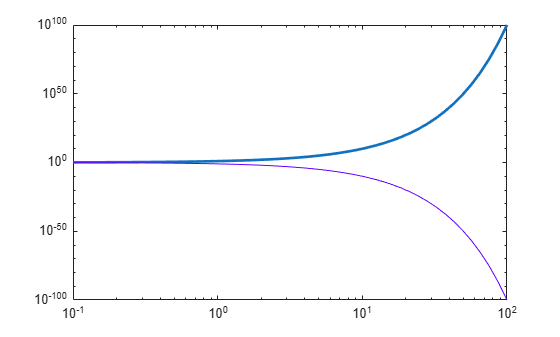## Input Arguments

collapse all

x-coordinates, specified as a scalar, vector, or matrix. The size and shape of `X` depends on the shape of your data and the type of plot you want to create. This table describes the most common situations.

Type of PlotHow to Specify Coordinates
Single point

Specify `X` and `Y` as scalars and include a marker. For example:

`loglog(1,2,'o')`

One set of points

Specify `X` and `Y` as any combination of row or column vectors of the same length. For example:

`loglog([1 2 3],[4; 5; 6])`

Multiple sets of points
(using vectors)

Specify consecutive pairs of `X` and `Y` vectors. For example:

`loglog([1 2 3],[4 5 6],[1 2 3],[7 8 9])`

Multiple sets of points
(using matrices)

If all the sets share the same x- or y-coordinates, specify the shared coordinates as a vector and the other coordinates as a matrix. The length of the vector must match one of the dimensions of the matrix. For example:

`loglog([1 2 3],[4 5 6; 7 8 9])`
If the matrix is square, `loglog` plots one line for each column in the matrix.

Alternatively, specify `X` and `Y` as matrices of equal size. In this case, `loglog` plots each column of `Y` against the corresponding column of `X`. For example:

`loglog([1 2 3; 4 5 6],[7 8 9; 10 11 12])`

`loglog` might exclude coordinates in some cases:

• If the coordinates include positive and negative values, only the positive values are displayed.

• If the coordinates are all negative, all of the values are displayed on a log scale with the appropriate sign.

• Zero values are not displayed.

Data Types: `single` | `double` | `int8` | `int16` | `int32` | `int64` | `uint8` | `uint16` | `uint32` | `uint64`

y-coordinates, specified as a scalar, vector, or matrix. The size and shape of `Y` depends on the shape of your data and the type of plot you want to create. This table describes the most common situations.

Type of PlotHow to Specify Coordinates
Single point

Specify `X` and `Y` as scalars and include a marker. For example:

`loglog(1,2,'o')`

One set of points

Specify `X` and `Y` as any combination of row or column vectors of the same length. For example:

`loglog([1 2 3],[4; 5; 6])`

Multiple sets of points
(using vectors)

Specify consecutive pairs of `X` and `Y` vectors. For example:

`loglog([1 2 3],[4 5 6],[1 2 3],[7 8 9])`

Multiple sets of points
(using matrices)

If all the sets share the same x- or y-coordinates, specify the shared coordinates as a vector and the other coordinates as a matrix. The length of the vector must match one of the dimensions of the matrix. For example:

`loglog([1 2 3],[4 5 6; 7 8 9])`
If the matrix is square, `loglog` plots one line for each column in the matrix.

Alternatively, specify `X` and `Y` as matrices of equal size. In this case, `loglog` plots each column of `Y` against the corresponding column of `X`. For example:

`loglog([1 2 3; 4 5 6],[7 8 9; 10 11 12])`

`loglog` might exclude coordinates in some cases:

• If the coordinates include positive and negative values, only the positive values are displayed.

• If the coordinates are all negative, all of the values are displayed on a log scale with the appropriate sign.

• Zero values are not displayed.

Data Types: `single` | `double` | `int8` | `int16` | `int32` | `int64` | `uint8` | `uint16` | `uint32` | `uint64`

Line style, marker, and color, specified as a string scalar or character vector containing symbols. The symbols can appear in any order. You do not need to specify all three characteristics (line style, marker, and color). For example, if you omit the line style and specify the marker, then the plot shows only the marker and no line.

Example: `"--or"` is a red dashed line with circle markers.

Line StyleDescriptionResulting Line
`"-"`Solid line`"--"`Dashed line`":"`Dotted line`"-."`Dash-dotted lineMarkerDescriptionResulting Marker
`"o"`Circle`"+"`Plus sign`"*"`Asterisk`"."`Point`"x"`Cross`"_"`Horizontal line`"|"`Vertical line`"square"`Square`"diamond"`Diamond`"^"`Upward-pointing triangle`"v"`Downward-pointing triangle`">"`Right-pointing triangle`"<"`Left-pointing triangle`"pentagram"`Pentagram`"hexagram"`HexagramColor NameShort NameRGB TripletAppearance
`"red"``"r"``[1 0 0]``"green"``"g"``[0 1 0]``"blue"``"b"``[0 0 1]``"cyan"` `"c"``[0 1 1]``"magenta"``"m"``[1 0 1]``"yellow"``"y"``[1 1 0]``"black"``"k"``[0 0 0]``"white"``"w"``[1 1 1]`Source table containing the data to plot, specified as a table or a timetable.

Table variables containing the x-coordinates, specified using one of the indexing schemes from the table.

Indexing SchemeExamples

Variable names:

• `"A"` or `'A'` — A variable called `A`

• `["A","B"]` or `{'A','B'}` — Two variables called `A` and `B`

• `"Var"+digitsPattern(1)` — Variables named `"Var"` followed by a single digit

Variable index:

• An index number that refers to the location of a variable in the table.

• A vector of numbers.

• A logical vector. Typically, this vector is the same length as the number of variables, but you can omit trailing `0` or `false` values.

• `3` — The third variable from the table

• `[2 3]` — The second and third variables from the table

• `[false false true]` — The third variable

Variable type:

• `vartype("categorical")` — All the variables containing categorical values

The table variables you specify can contain any numeric values. However, `loglog` might exclude negative and zero values from the plot in the same way as it does when you specify `X` and `Y` as vectors containing negative or zero values.

If `xvar` and `yvar` both specify multiple variables, the number of variables must be the same.

Example: `loglog(tbl,["x1","x2"],"y")` specifies the table variables named `x1` and `x2` for the x-coordinates.

Example: `loglog(tbl,2,"y")` specifies the second variable for the x-coordinates.

Example: `loglog(tbl,vartype("numeric"),"y")` specifies all numeric variables for the x-coordinates.

Table variables containing the y-coordinates, specified using one of the indexing schemes from the table.

Indexing SchemeExamples

Variable names:

• `"A"` or `'A'` — A variable called `A`

• `["A","B"]` or `{'A','B'}` — Two variables called `A` and `B`

• `"Var"+digitsPattern(1)` — Variables named `"Var"` followed by a single digit

Variable index:

• An index number that refers to the location of a variable in the table.

• A vector of numbers.

• A logical vector. Typically, this vector is the same length as the number of variables, but you can omit trailing `0` or `false` values.

• `3` — The third variable from the table

• `[2 3]` — The second and third variables from the table

• `[false false true]` — The third variable

Variable type:

• `vartype("categorical")` — All the variables containing categorical values

The table variables you specify can contain any numeric values. However, `loglog` might exclude negative and zero values from the plot in the same way as it does when you specify `X` and `Y` as vectors containing negative or zero values.

If `xvar` and `yvar` both specify multiple variables, the number of variables must be the same.

Example: `loglog(tbl,"x",["y1","y2"])` specifies the table variables named `y1` and `y2` for the y-coordinates.

Example: `loglog(tbl,"x",2)` specifies the second variable for the y-coordinates.

Example: `loglog(tbl,"x",vartype("numeric"))` specifies all numeric variables for the y-coordinates.

Target axes, specified as an `Axes` object. If you do not specify the axes and if the current axes is Cartesian, then `loglog` uses the current axes.

### Name-Value Arguments

Specify optional pairs of arguments as `Name1=Value1,...,NameN=ValueN`, where `Name` is the argument name and `Value` is the corresponding value. Name-value arguments must appear after other arguments, but the order of the pairs does not matter.

Before R2021a, use commas to separate each name and value, and enclose `Name` in quotes.

Example: `loglog([1 2],[3 4],'Color','red')` specifies a red line for the plot.

Note

The properties listed here are only a subset. For a complete list, see Line Properties.

Color, specified as an RGB triplet, a hexadecimal color code, a color name, or a short name. The color you specify sets the line color. It also sets the marker edge color when the `MarkerEdgeColor` property is set to `'auto'`.

For a custom color, specify an RGB triplet or a hexadecimal color code.

• An RGB triplet is a three-element row vector whose elements specify the intensities of the red, green, and blue components of the color. The intensities must be in the range `[0,1]`, for example, ```[0.4 0.6 0.7]```.

• A hexadecimal color code is a string scalar or character vector that starts with a hash symbol (`#`) followed by three or six hexadecimal digits, which can range from `0` to `F`. The values are not case sensitive. Therefore, the color codes `"#FF8800"`, `"#ff8800"`, `"#F80"`, and `"#f80"` are equivalent.

Alternatively, you can specify some common colors by name. This table lists the named color options, the equivalent RGB triplets, and hexadecimal color codes.

Color NameShort NameRGB TripletHexadecimal Color CodeAppearance
`"red"``"r"``[1 0 0]``"#FF0000"``"green"``"g"``[0 1 0]``"#00FF00"``"blue"``"b"``[0 0 1]``"#0000FF"``"cyan"` `"c"``[0 1 1]``"#00FFFF"``"magenta"``"m"``[1 0 1]``"#FF00FF"``"yellow"``"y"``[1 1 0]``"#FFFF00"``"black"``"k"``[0 0 0]``"#000000"``"white"``"w"``[1 1 1]``"#FFFFFF"``"none"`Not applicableNot applicableNot applicableNo color

Here are the RGB triplets and hexadecimal color codes for the default colors MATLAB uses in many types of plots.

`[0 0.4470 0.7410]``"#0072BD"``[0.8500 0.3250 0.0980]``"#D95319"``[0.9290 0.6940 0.1250]``"#EDB120"``[0.4940 0.1840 0.5560]``"#7E2F8E"``[0.4660 0.6740 0.1880]``"#77AC30"``[0.3010 0.7450 0.9330]``"#4DBEEE"``[0.6350 0.0780 0.1840]``"#A2142F"`Line width, specified as a positive value in points, where 1 point = 1/72 of an inch. If the line has markers, then the line width also affects the marker edges.

The line width cannot be thinner than the width of a pixel. If you set the line width to a value that is less than the width of a pixel on your system, the line displays as one pixel wide.

Marker size, specified as a positive value in points, where 1 point = 1/72 of an inch.

Marker outline color, specified as `"auto"`, an RGB triplet, a hexadecimal color code, a color name, or a short name. The default value of `"auto"` uses the same color as the `Color` property.

For a custom color, specify an RGB triplet or a hexadecimal color code.

• An RGB triplet is a three-element row vector whose elements specify the intensities of the red, green, and blue components of the color. The intensities must be in the range `[0,1]`, for example, ```[0.4 0.6 0.7]```.

• A hexadecimal color code is a string scalar or character vector that starts with a hash symbol (`#`) followed by three or six hexadecimal digits, which can range from `0` to `F`. The values are not case sensitive. Therefore, the color codes `"#FF8800"`, `"#ff8800"`, `"#F80"`, and `"#f80"` are equivalent.

Alternatively, you can specify some common colors by name. This table lists the named color options, the equivalent RGB triplets, and hexadecimal color codes.

Color NameShort NameRGB TripletHexadecimal Color CodeAppearance
`"red"``"r"``[1 0 0]``"#FF0000"``"green"``"g"``[0 1 0]``"#00FF00"``"blue"``"b"``[0 0 1]``"#0000FF"``"cyan"` `"c"``[0 1 1]``"#00FFFF"``"magenta"``"m"``[1 0 1]``"#FF00FF"``"yellow"``"y"``[1 1 0]``"#FFFF00"``"black"``"k"``[0 0 0]``"#000000"``"white"``"w"``[1 1 1]``"#FFFFFF"``"none"`Not applicableNot applicableNot applicableNo color

Here are the RGB triplets and hexadecimal color codes for the default colors MATLAB uses in many types of plots.

`[0 0.4470 0.7410]``"#0072BD"``[0.8500 0.3250 0.0980]``"#D95319"``[0.9290 0.6940 0.1250]``"#EDB120"``[0.4940 0.1840 0.5560]``"#7E2F8E"``[0.4660 0.6740 0.1880]``"#77AC30"``[0.3010 0.7450 0.9330]``"#4DBEEE"``[0.6350 0.0780 0.1840]``"#A2142F"`Marker fill color, specified as `"auto"`, an RGB triplet, a hexadecimal color code, a color name, or a short name. The `"auto"` option uses the same color as the `Color` property of the parent axes. If you specify `"auto"` and the axes plot box is invisible, the marker fill color is the color of the figure.

For a custom color, specify an RGB triplet or a hexadecimal color code.

• An RGB triplet is a three-element row vector whose elements specify the intensities of the red, green, and blue components of the color. The intensities must be in the range `[0,1]`, for example, ```[0.4 0.6 0.7]```.

• A hexadecimal color code is a string scalar or character vector that starts with a hash symbol (`#`) followed by three or six hexadecimal digits, which can range from `0` to `F`. The values are not case sensitive. Therefore, the color codes `"#FF8800"`, `"#ff8800"`, `"#F80"`, and `"#f80"` are equivalent.

Alternatively, you can specify some common colors by name. This table lists the named color options, the equivalent RGB triplets, and hexadecimal color codes.

Color NameShort NameRGB TripletHexadecimal Color CodeAppearance
`"red"``"r"``[1 0 0]``"#FF0000"``"green"``"g"``[0 1 0]``"#00FF00"``"blue"``"b"``[0 0 1]``"#0000FF"``"cyan"` `"c"``[0 1 1]``"#00FFFF"``"magenta"``"m"``[1 0 1]``"#FF00FF"``"yellow"``"y"``[1 1 0]``"#FFFF00"``"black"``"k"``[0 0 0]``"#000000"``"white"``"w"``[1 1 1]``"#FFFFFF"``"none"`Not applicableNot applicableNot applicableNo color

Here are the RGB triplets and hexadecimal color codes for the default colors MATLAB uses in many types of plots.

`[0 0.4470 0.7410]``"#0072BD"``[0.8500 0.3250 0.0980]``"#D95319"``[0.9290 0.6940 0.1250]``"#EDB120"``[0.4940 0.1840 0.5560]``"#7E2F8E"``[0.4660 0.6740 0.1880]``"#77AC30"``[0.3010 0.7450 0.9330]``"#4DBEEE"``[0.6350 0.0780 0.1840]``"#A2142F"`## Tips

• Use `NaN` or `Inf` to create breaks in the lines. For example, this code plots a line with a break between `y=2` and `y=4`.

` loglog([1 2 3 4 5],[1 2 NaN 4 5])`

• The `loglog` function uses colors and line styles based on the `ColorOrder` and `LineStyleOrder` properties of the axes. `loglog` cycles through the colors with the first line style. Then, it cycles through the colors again with each additional line style.

You can change the colors and the line styles after plotting by setting the `ColorOrder` or `LineStyleOrder` properties on the axes. You can also call the `colororder` function to change the color order for all the axes in the figure.

## Algorithms

The `loglog` function plots coordinates on a log scale by setting the `XScale` and `YScale` properties of the axes to `'log'`. However, if the axes `hold` state is `'on'` before you call `loglog`, those properties do not change, and the plot might display on a linear or semilog scale.

## Version History

Introduced before R2006a

expand all